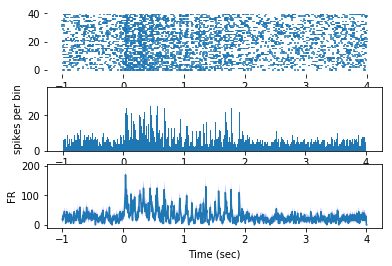In :
# -*- coding: utf-8 -*-
"""
Created on Sun Oct 27 14:31:30 2019

@author: Healey (Luke Remage-Healey)
"""
import os
import matplotlib.pyplot as plt
import numpy as np
import matplotlib
matplotlib.rcParams['font.size'] = 18.0
import pandas as pd
import csv

"""this is a routine to take kilosort sorted spikes (timestamps in '.txt' files)
and trigger them with a stimulus key (typically a '.csv' format) to generate a raster, PSTH,
and inst FR plot for a given stimulus. beware, this code is quite nested and slow...."""

Out:
"this is a routine to take kilosort sorted spikes (timestamps in '.txt' files)\nand trigger them with a stimulus key (typically a '.csv' format) to generate a raster, PSTH, \nand inst FR plot for a given stimulus. beware, this code is quite nested and slow...."
In :
def key(csvfile, start, end):
"""returns the timestamps of the kilosort  stimulations in 'csvfile' as a dict
with the restriction of the 'start' and 'end' times of the recording"""
#    """and all values are multiplied 1000x"""
t=[]
#    d=[]

with open(csvfile,'r') as myfile:
t.append(float(row))
#            d.append(float(row))

temp = []
for i in range(len(t)):
if t[i] < start:
continue
if t[i] > end:
continue
else:
temp.append(t[i]) #, d[i]])
#    """sets up a dict for accepting the key times wth correct identifiers, depending on the column"""
#    a ={}
#    for i in range(len(temp)):
#        a[temp[i]]=[]
#
#    """populates the a dict with key timestamps"""
#    for i in range(len(temp)):
#        a[temp[i]].append(int(float(temp[i])))

return temp

In :
def readspikes(spikes):

"""reads in a '.txt' kilosorted spike train into format for plotting"""
myfile=open(spikes,'r')
myfile.close()

#convert txt into a list (do anything on the r in that one argument)

#to make numbers work:
spks = [float(i) for i in gadsplit]

return spks


In :
def raster_H_FR(keys, clus, clusname, start, end, ras_start, ras_end):
'''Takes a set of key triggers 'keys', a sorted spike cluster 'clus', the name of the cluster
for plotting on the figure 'clusname' as a string, the 'start' and
'end' of a segment of recording, and the frames for the raster start 'ras_start' and
raster end 'ras_end', to generate a peristimulus raster histogram, and FR plot on the same plot'''

# below generates the rasterized spikes
a = {}
for i in keys:
temp = []
for j in clus:
if j > start:
if j < end:
if j > i-ras_start:
if j < i + ras_end:
temp.append(j-i)
a[i] = temp
s = pd.Series(a)   #this above generates the raster as a pandas data series

#below generates the histogram
bins=[]
for i in np.arange(-ras_start, ras_end+0.01, 0.005):   #to use arange, which allows decimals
bins.append(round(i,3))     #to round the float to 2 decimal places
hist = {}
for i in bins:
hist[i] = 0

for i in s.index:
for j in s[i]:
for k in bins:
if j < min(bins):
break
if j > max(bins):
break
if j > k and j < round(k+.005, 3):
hist[round(k+.005,3)] += 1

#below generates the instantaneous FR plot
b = {}
for i in a.keys():
b[i] = {}
for k in bins:
b[i].update({k:0})
temp = 0
for j in a[i]:
if j < min(bins):
break

if j > max(bins):
break
if j > k and j < round(k+0.005, 3):
temp += 1
b[i].update({k:temp/0.005})

FR = pd.DataFrame()

for i in b.keys():
FR[i] = b[i].values()
FR['ave'] = np.mean(FR, axis=1)
FR['std'] = np.std(FR, axis=1)
FR['sterr'] = np.std(FR, axis=1)/np.sqrt(len(FR.columns))
FR['x'] = hist.keys()

#below plots all three on the same axes
f = plt.figure('' + str(clusname) + '')
plt.subplot(3,1,1)       #pos is a three digit integer, where the first digit is the number of rows, the second the number of columns, and the third the index of the subplot.
plt.eventplot(s)    #to plot the raster
plt.box(False)
#    plt.xaxis(False)

plt.subplot(3,1,2)
plt.bar(hist.keys(), hist.values(), width=0.01, label = 'hist') #to plot the PSTH
#plt.ylim(0, int(max(hist.values()))+5)

plt.ylabel('spikes per bin')

#plt.legend()

plt.subplot(3,1,3) #to plot the instantaneous FR
plt.plot(FR['x'], FR['ave'])
plt.fill_between(FR['x'], (FR['ave']+FR['sterr']), (FR['ave']-FR['sterr']), facecolor='blue', alpha=0.5)
plt.ylabel('FR')
plt.xlabel('Time (sec)')

plt.show()

return FR

In :
clus238 = readspikes('73019_L_site5_SU_cluster238.txt')

In :
key2 = key('audio_stimulus_key.csv', 0, 3000)

In :
len(key2)

Out:
40
In :
"""just a check to make sure it's all good"""

Out:
"just a check to make sure it's all good"
In :
test2382 = raster_H_FR(key2, clus238, 'cl238', 0, 3000, 1, 4)In :
print("VOILA!")

VOILA!

In [ ]: